﻿ 用光照法无损检测鸡蛋煮熟程度

# 用光照法无损检测鸡蛋煮熟程度Illumination Method for Non-Invasive Detection of Egg’s Boiled Degree

Abstract: An egg’s boiled degree is always estimated and controlled qualitatively by experience in daily lives; however, when it needs quantitative measurement and precise control, the common methods have their limitation. An illumination method for non-invasive detection of an egg’s boiled degree is proposed in this paper. Due to the protein denaturation of egg while being boiled, its luminousness gradually changes when illuminated by LED lamp. By photographing the egg and analyzing the grayscale of the photos, the egg’s central temperature and boiled degree can be determined and controlled quantitatively. This method may be generalized to monitoring some solid-liquid conversion process.

1. 引言

2. 理论分析

2.1. 鸡蛋热传导模型

${\nabla }^{\text{2}}T=\frac{1}{K}\frac{\partial T}{\partial t}$ (1)

$K=\frac{k}{\rho c}$ (2)Table 1. Parameters of egg yolk and egg white

$\left\{\begin{array}{l}\frac{1}{{r}^{2}}\frac{\partial }{\partial r}\left({r}^{2}\frac{\partial T}{\partial r}\right)=\frac{1}{K}\frac{\partial T}{\partial t}\\ {T\left(r,t\right)|}_{t=0}={T}_{0}\\ {T\left(r,t\right)|}_{r=0}={T}_{0}\\ {T\left(r,t\right)|}_{r=R}={T}_{w}\end{array}$ (3)

$T\left(r,t\right)={T}_{w}-\frac{2R\left({T}_{w}-{T}_{0}\right)}{\text{π}r}\underset{n=1}{\overset{\infty }{\sum }}\left(\frac{{\left(-1\right)}^{n+1}}{n}\right)\mathrm{sin}\frac{n\text{π}r}{R}{\text{e}}^{-\frac{K{n}^{2}{\text{π}}^{2}t}{{R}^{2}}}$ (4)

${T}_{C}\left(t\right)=\underset{r\to 0}{\mathrm{lim}}T\left(r,t\right)={T}_{w}-2\left({T}_{w}-{T}_{0}\right)\underset{n=1}{\overset{\infty }{\sum }}{\left(-1\right)}^{n+1}{\text{e}}^{-\frac{K{n}^{2}{\text{π}}^{2}t}{{R}^{2}}}$ (5)

${T}_{C}\left(t\right)={T}_{w}-2\left({T}_{w}-{T}_{0}\right){\text{e}}^{-\frac{6000t}{9{R}^{2}}}$ (6)

2.2. 光照法原理

${I}_{t}={I}_{0}{\text{e}}^{-{a}_{t}l}$ (7)

${I}_{s}={I}_{0}{\text{e}}^{-{a}_{s}l}$ (8)

$I={I}_{\text{0}}{\text{e}}^{-al}$ (9)

$a={a}_{t}+{a}_{s}$ (10)

3. 实验测定鸡蛋熟度与透射光强定量关系

3.1. 实验整体流程

1、将一鸡蛋在98℃水中加热累计12分钟，每隔1分钟取出透光拍照并用数学软件得到中心灰度平均值，求出煮熟程度与灰度的关系趋势；

2、对蛋黄蛋清吸收系数单独分析，检验其透光度与厚度的关系是否满足光的吸收定律；

3、通过理论分析与测量数据综合给出不同参数鸡蛋(尺寸、蛋黄体积比、蛋壳颜色)的熟度与图像灰度值的关系。

3.2. 实验装置与步骤

1、实验装置(如图1示意)：

1) 强光手电筒：持续照度约为100,000 Lux；

2) 摄像设备：Casio® ZR1500相机；

3) 照度计：量程200,000 Lux，精度0.1 Lux。

2、操作步骤：

1) 用游标卡尺测量待测鸡蛋的长轴a与短轴b，取鸡蛋平均半径为 $R=\left(a+b\right)/4$

2) 将蛋壳颜色与比色卡对比读出对应颜色系数α；

3) 将鸡蛋水平置于装置凹槽中，调整相机使鸡蛋位于镜头中心，固定相机参数为ISO：1600；快门速度：1/30 s；放大倍率：x2.3；光圈大小：f4.5；

4) 拍摄鸡蛋并将图像导入Matlab®分析得到中心灰度g与鸡蛋平均半径R和蛋黄半径r的关系。

3.3. 参数标定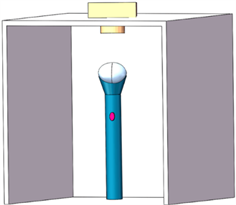Figure 1. Schematic diagram of the experimental device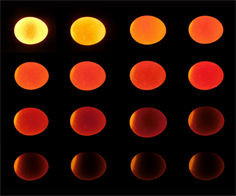Figure 2. The photographs of an egg in different boiled duration of time. The boiled duration of the first photograph is 1 minute and increases by 1 minute in each photograph from left to right, top to bottom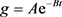(11)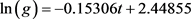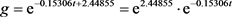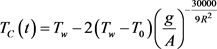(12)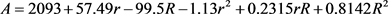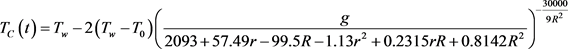(13)

4. 灵敏度与有效性检测

4.1. 灵敏度检测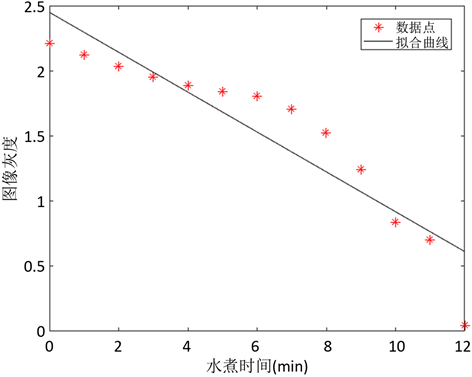Figure 3. The fitting curve of boiled duration of time and grayscale of the egg’s central part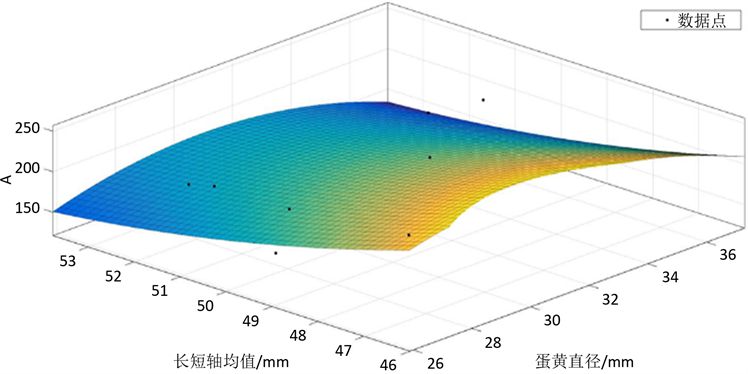Figure 4. The fitting surface of parameter A and the diameters of the egg and the yolk(14)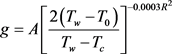(15)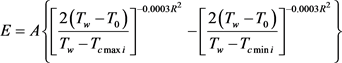(16)

4.2. 有效性验证

1) 选取多个鸡蛋按3.2步骤测量并将所得参数带入(12)式计算得到理论中心温度；

2) 将探头式温度计插入鸡蛋测量实际中心温度；

3) 将理论温度与实际温度进行比较并计算误差。

5. 产品化设计Table 2. The theoretical and actual central temperature of the egg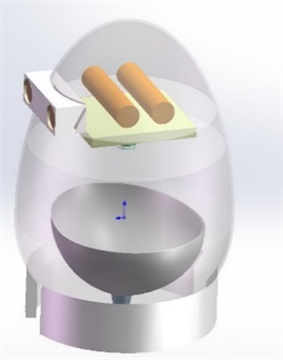Figure 5. Three-dimensional rendering for conceptual design of an adjustable egg cooker

6. 结论

 王栓巧, 郁志宏. 基于机器视觉鸡蛋品质检测机构的研究[J]. 机械设计与制造, 2009(12): 84-86.

 金志强, 张锦胜. 利用核磁共振及成像原理研究鸡蛋的煮熟过程[J]. 食品工业科技, 2008, 29(8): 112-114.

 Problems of IYPT.
http://archive.iypt.org/problems/

 Kemps, B.J. and Bamelis, F.R. (2006) Visible Transmission Spec-troscopy for the Assessment of Egg Freshness. Journal of the Science of Food & Agriculture, 86, 1399-1406.

 Durarte, F.J. (1979) Heat Diffusion in a Solid Sphere and Fourier Theory, an Elementary Practical Example. American Journal of Physics, 47, 981-983.

 Foong, S.K. (2006) How Long Does It Take to Boil an Egg? European Journal of Physics, 27, 119-131.

Top